Edward Nightingale is a master woodworker and woodcarver with over 30 years of experience using the tools of the trade, measure and geometry, as well he has been a performing musician for over 30 years. Applying his understanding of geometry, measure, mathematics and music, he has discovered designed in the geometry and layout of the pyramid complex at Giza, by the original architects, high science and mathematics. One of the mysteries solved in The Giza Template is the root of the measuring system, how the original numbers are derived and their relationship to the speed of light. See Edward’s website at: http://www.thegizatemplate.com

#### More articles

The Geometry of Giza by Edward Nightingale, 19 February 2011

After presenting my work in the article The Geometry of Giza the question of intent of the builders of Giza to purposely encode information into the complex was raised. The E-book would have addressed the valid questions posed in the forum, but unfortunately is behind schedule. Graham has been gracious enough to allow me to address some of the questions in a separate article. I have assembled some of the information to show the builders intent to encode this specific knowledge within the design of the Giza Complex.

I am presenting a design based on simple geometry that can be interpreted using only simple tools, a compass and straight edge. The approximate geometric values can then be corrected to their exact values using the number system and mathematics encoded.

I hypothesize the “intended dimensions” used by the designers for the base lengths of the three main pyramids and the Sphinx are as follows:

 Khufu base length 440 Royal Cubits 441 Root Cubits 756 ft Khafre base length 411 Royal Cubits 706.1727 ft Menkaure base length #1 200 Royal Cubits 343.6363 ft Menkaure base length #2 195 Royal Cubits 335.0454 ft Sphinx length 137 Royal Cubits 235.3908 ft The exact circle diameter is 3699 Royal Cubits 6355.554 ft

1 Root Cubit equal 20.57142 inches
1 Royal Cubits equal 20.61818 inches
These values are found encoded within this template and are covered in the following article which picks up from the end of the first article, The Geometry of Giza.

Addressing every point of this research is beyond the scope of two articles, I am doing the best I can to have the E-Book available. I hope this information will help answer the many questions this work has generated.

## The 8, 9 and 11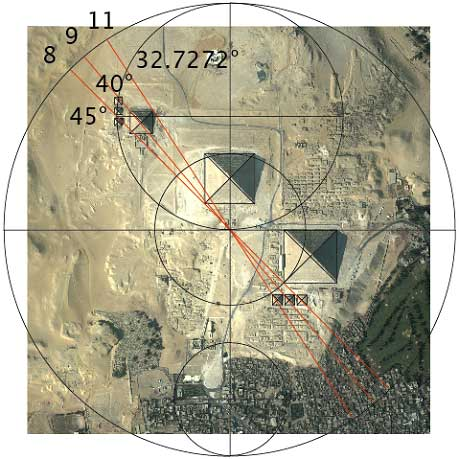We were able to locate the three main pyramids, Khufu, Khafre, and Menkaure using the three satellite pyramids of Khufu and the axis of the main circle. It seems that at least one of the functions of the satellite pyramids of Khufu were to geometrically locate the pyramids and create the angles of 45°, 40°, 32.7272° which can divide the circle by 8, 9, and 11. We will address the number 11 and 32.7272 at a later point, for now we will look at the importance of the 8 and 9.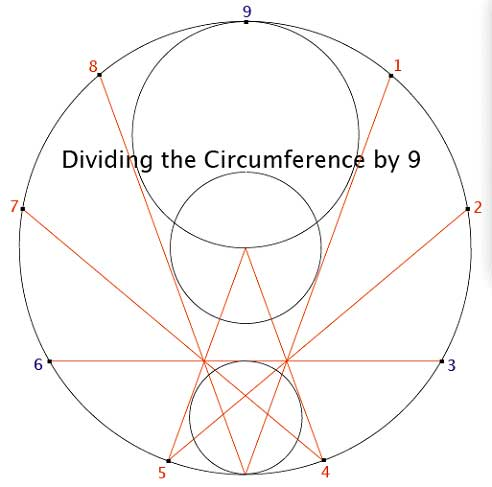Using the 4-3-2- geometry a straightedge and the 3 circles of ¼, ?, and ½ proportions of the main circle as guides we are able divide the circumference of the circle by 9. This would tend to support our hypothesis that the angled line of 40° dividing the circumference by 9. I think its logical to conclude that use of this specific geometry was intended by the original designers (among other things) to divide the circumference of the circle by 9. Take a few moments and look closely at this geometry and ask yourself what famous symbol does it remind you of? We will discuss that at a later point. We will also cover the mathematics of a circle divided by 9, but for now let’s see if the number 8 has any relationship to the circle divided by 9.

For more information on 9 based Vortex mathematics visit www.markorodin.com and www.love528.com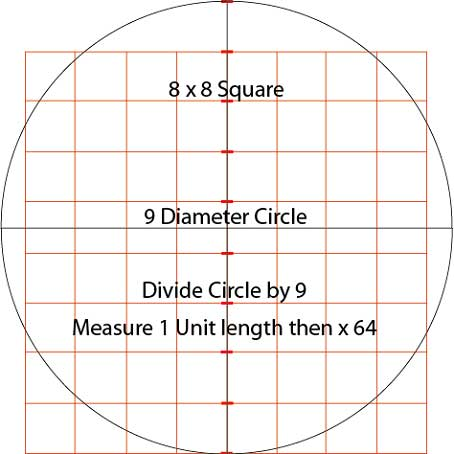The Egyptians used a simple mathematical equation to approximate the area of a circle, with a compass they divided the diameter of the circle into 9 equal units, measured the length of 1 unit with any standard of measure and multiplied that number by 64, so if we have a circle measuring 9 inches in diameter you multiply 1 inch by 64 and have 64 square inches of area in the circle.

Comparing the previous approximating calculation to determining the actual area of a circle using Pi of 3.142857, (or 22/7) the calculation would be; Pi x R2 = Area, so 3.142857 x 4.5(radius of 9 diameter circle) = 14.1428565 x 4.5. = 63.6428. The approximation of the area of the circle is 64 using the 8 square and 63.6428 using Pi. So the calculation to find area of a circle with Diameter 9 approximating 8 Square seems quite practical.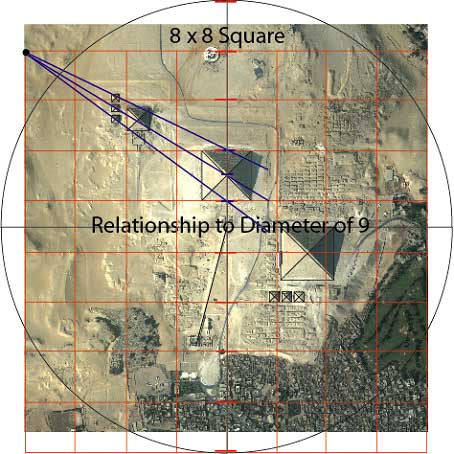Placing an 8 x 8 grid over our 9 diameter circle in the manner above and using the upper left hand corner of the square as a point, we draw angled lines through the center of the satellite pyramids of Menkaure just as we did with the satellite pyramids of Khufu to determine the angled lines and the 8, 9 and 11 numbers. Notice that the three angled lines intersect the divisions of the diameter of the circle in the 6th position up from the bottom where our initial determination of the diameter division of 9 is located, confirming the 8 x 8 square. The satellite pyramids of Khufu are associated with the circle and its axis and the satellite pyramids of Menkaure are associated with the square and its corner confirming this 8, 9 relationship.

The Egyptian accounts of the world’s creation differ depending on the city. There are accounts from the cities of Heliopolis, Hermopolis, Memphis and Thebes, each with its particular God. These separate stories seem to be differing aspects of creation itself. We will revisit in more detail the 9 Ennead and 8 Ogdoad at a later point and see how these stories are associated with the science encoded at Giza.

The Egyptians Gods were the 9 Ennead and 8 Ogdoad. The Ennead was a group or council of 9 Gods. They were Atum, Shu Tefnut, Geb, Set, Nut, Isis, Osirus, and Nephthys. The word ennead is defined as “a group or set of 9”.

The Ogdoad are defined as “a group or set of 8” each one having a masculine and feminine pair and represents the chaos out of which the world was created. The 8 Ogdoad or 4 male, female pair were Nun and Naunet representing water, Heh and Hauhet representing infinity, Amun and Amaunet invisibility, and Kek and Kauket representing darkness. These four fundamental forces were unbalanced and lead to the creation of Ra, the Sun. By working together with the four forces they created all things.

## The Eye of Ra = 63/64ths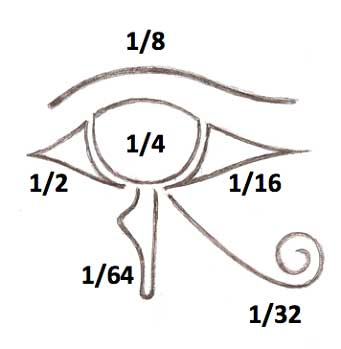The Eye of Ra or Horus is composed of 6 parts or glyphs that make up the eye. Each part represents a fraction, ½, ¼, ?, 1/16, 1/32, and 1/64. If we add these 6 fractions we get 63/64. The fraction 63/64 is a clue here I suspect to impart to the initiate the 8 square and 9 circle (among other things). This symbol has other meanings as well and will be covered at a later point. For our discussion here we will use this as a fractional glyph. So with the association of The Eye of Ra with the 8, 9 Square and Circle, we have to consider that there are possibly deeper meanings or uses of the circle and square above the use of an equation to determine the area of a circle.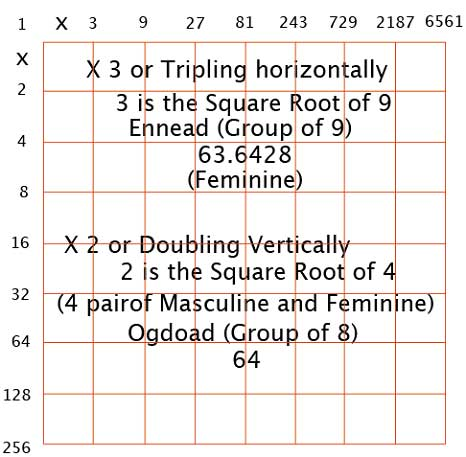If we combine the aspects of the Circle divided by 9, the Ennead, with the aspects of the 8 (4 pair) side of the Square, the Ogdoad, then take the square roots of each, 3 is the square root of 9 and 2 is the square root, 4 (pair) and use as a doubling sequence down the X axis, or the left vertical column, and a tripling sequence across the Y axis or top horizontal column, we create a grid of 2 separate sequences that combine by multiplying one column by the other and arriving with the sum at the intersections. These two aspects, the 2 can be seen as Masculine, the Square of 64 and 3 as Feminine, and the Circle of 63.6428.

Looking back on what we have uncovered so far by following the numbers left to us as clues, we’ve looked at what those two numbers, 8 and 9 represented in the Egyptian mythology, and can see the connections of the mythology to our 8 x 8 Square and the 9 Diameter circle. It’s important to note that these numbers are also very relevant with similar meanings in many different cultures from around the world, we will look into this at a later point.

So we have just introduced three new numbers into our 8 x 8 grid, the numbers 1, 2 and 3. Let’s see what the ancient mythologies, in particular ancient Egyptian say about these three numbers. The number 1 is associated with or represented by the Wadjet or Ujat, the “Whole One”, The Eye of Ra and All Seeing Eye. This seems to coincide with our use of the Eye of Ra to represent the 63/64ths with the circle and the square, the feminine and the masculine.

The number 2 in Egyptian mythology represents the duality of the male and female and is the essence of the 4 “pair” or 8 Ogdoad.

The number 3 is associated with the Egyptian Hermetic traditions of Thoth, Thrice Great, or the Supreme Power. The number three or a trinity being at the root of many different cultural belief systems and are not easily overlooked here, we begin seeing a pattern of these same numbers all pointing towards similar symbolical meanings. It seems that the balanced combining of the 2, the Masculine and the 3 the Feminine are critical in obtaining 1 or the “Whole One”.

There is another source that we can find that confirms our use of the square 8×8 grid using the doubling (x2) and tripling (x3) numbers and comes from Plato in his work, Timeus and is explaining how God created man, this is an excerpt from that explanation;

“He first marked off a section of the whole (1), and then another twice the size of the first; (2) next a third, half as much as the second and three times the first, (3), a fourth twice the size of the second, (4) a fifth three times the third, (9) a sixth eight times the first, (8) a seventh twenty-seven times the first.” (27)

Pythagoras also is a source to confirm this number sequence known as the “Lambda number sequence” 1, 2, 3, 4, 9, 8, and 27, also known to the Pythagoreans as the “Tetrad” and is written in a triangular form, the shape of the Lambda that is the 11th letter of the Greek alphabet and we will discuss the number 11 at a later point. There is much more to discuss here but is beyond the scope of this chapter, for now I am showing the use of this particular numbering sequence in history.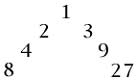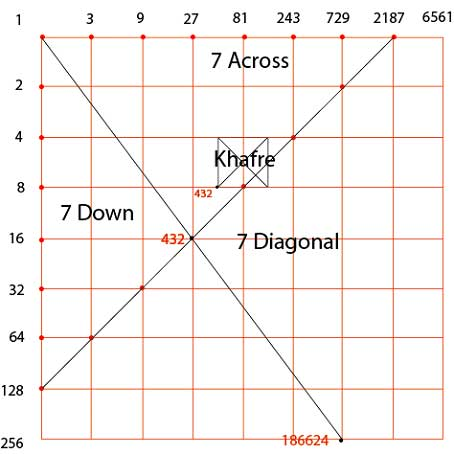### The Pythagorean triangle

Following the same train of thought that specific numbers are encoded we look on our grid to see if the number 432 is on the grid and as we can see it is located at the
16 x 27 intersection with a secondary point at 8 x 54. Using musical terms, the main 432 is the 4th “note” and 3rd “interval” on a diagonal line of 7 “intervals” created by 7 “intervals” across the top and 7 “intervals” down the left side of the grid. An “interval” is the space between one “note” to the next, and the red dots are the “notes”. Several very interesting things are going on here, first the number 432 is on a point on the grid of 4 down and 3 over or 4/3, a Pythagorean 3, 4, 5, Triangle, this creates an angle of 53.13° degrees, the angle of the Pyramid of Khafre. There is a secondary number of 432 on the grid as well and is located exactly on the South Eastern corner of the pyramid of Khafre when the grid is overlaid on the plan view of the pyramid complex. If we draw the angled line from the 1 on the grid intersecting the 432 and continue to the 8 down and 6 across or 8/6 we have a very interesting number, 186624, it is 432 squared or 432 x 432 = 186624. This number is also very close to the exact speed of light, 186282 miles per second, I call it the Root speed of light. So we can see that the pyramid of Khafre is representing the Pythagorean 3,4,5 Triangle and creates a connection between the numbers 432 and 186624. We also have introduced three numbers to our grid, three 7’s. Let’s take a look at the number 7 in mythology and see if it relates to what we have found in our grid.

In Egyptian mythology there are 7 Hathors that have 7 jars in 7 tunics. Ra has 7 hawks representing the 7 Wise Ones. 6 cows and 1 bull represent fertility. There are 7 houses of the underworld, with 3 times 7 gates. The number 7 is the sacred number of Osiris. This mythology matches exactly what we have found on the grid, three 7’s. There are many references to the number 7 in all cultures and without much further discussion here we can feel certain that the three 7’s on the grid is very significant.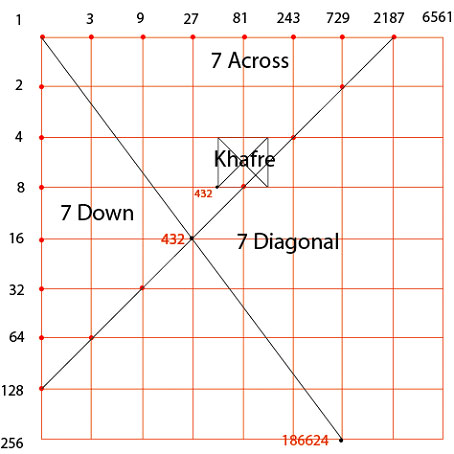## “Menkaure is Divine”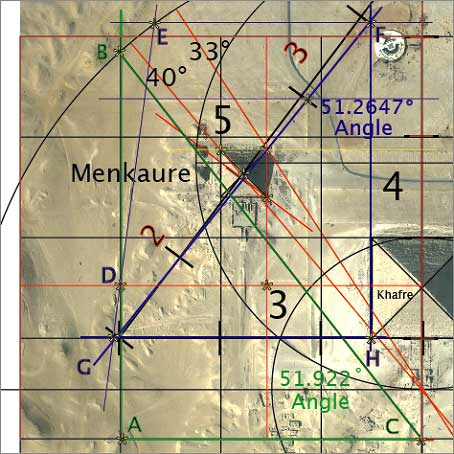Menkaure, the third pyramid I propose is designed to “bring together” the circle (9) and the square (8) while directing us to the 7 x 7 x 7, the (2) doubling and (3) tripling down and across the X and Y axis.

Let’s take a close look at the angles of Menkaure. First I want to note here that I use the two base lengths of Menkaure given as 343 ft and 335 ft by Mark Lenher in his book The Complete Pyramids which I believe he is getting from the Giza Mapping Project and is not available to the public as far as I know. The reasons I use his numbers are they fit exactly into a coherent, logical plan where the Petrie and Cole surveys seem to have difficulty in determining the exact corners of Menkaure due to its rough condition, it goes without saying I would love to have the GMP survey to corroborate this measurement. I don’t feel Mark Lehner would give the measurements as 343 ft x 335 ft without a good reason for doing so, and according to one of the engineers from the GMP it is accurate to a gnats eyelash.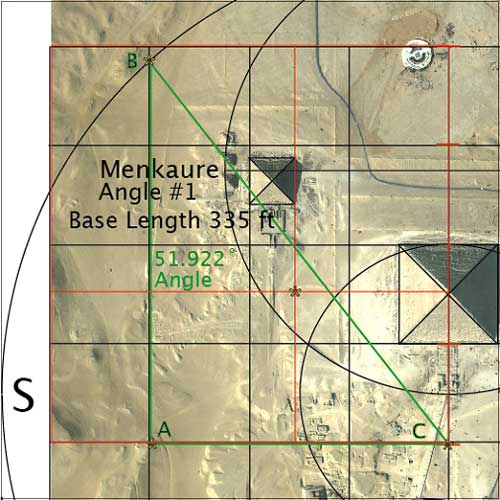The dimensions I use for Menkaure are as follows; base length #1 343 ft, #2 335 ft and a height 4/9ths of Khufu equaling 213.796 ft. With that being said, we focus on the upper left hand corner of our 8×8 grid. Using ¼ of the grid, a 4×4 we divide it at its Phi ratio point (the orange square with Phi division). Drawing a vertical line (in green) from point A using the first grid line in from the left to B where it intersects the circle, then a line from B to C create an angle of 51.922°. If we do the math for a pyramid with a base length of 335, a height of 213.796 we get an angle of 51.922° and interestingly 7,997,790 (three 7s and three 9s) cubic ft in volume. The key numbers here are the volume consisting of three 7s, and three 9s. Next let’s see what the 343 ft base length reveals.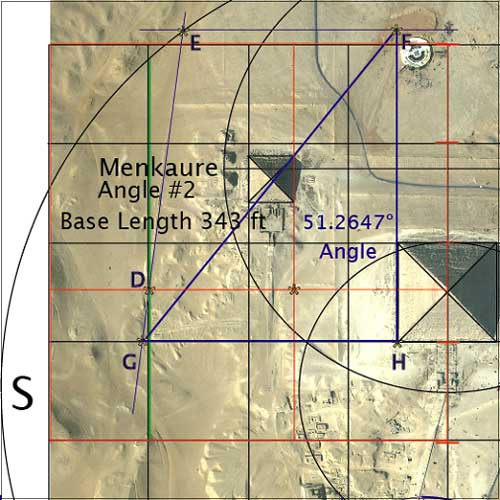The base length of 343 ft is revealing in itself as the number 343 equals 7 x 7 x 7 = 343. This angle explains the reason for the angled wall structure to the South of Menkaure, the light blue line staring from point D, the intersection of the Phi ratio line and the green vertical grid line. Drawing the line West from D to E where it intersects the circle and East to G where it intersects with grid line creates the angle of the wall structure. Drawing a horizontal line from point E North to point F that is a grid line in the 9 x 9 square (not shown here for clarity) as well as the Southern base edge of Khafre to point H. An angled line in dark blue from point F to point G form the angle of 51.2647°. The mathematical equation for a pyramid with a base length of 343 ft and height of 213.796 ft is an angle of 51.2647° and a volume of 8,384,330 cubic ft. The key numbers here are three 7s.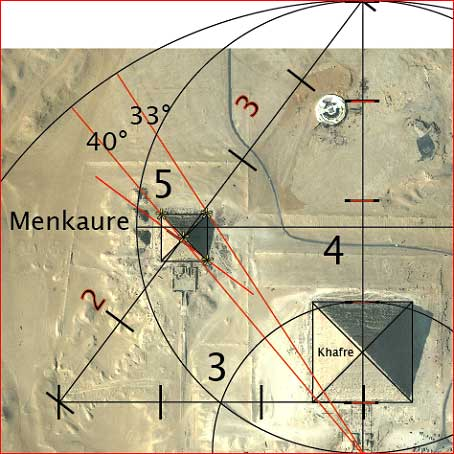The relationship of Menkaure to Khufu is it is 4/9ths Khufus height, 213.796 ft. The relationship to Khafre is shown above. The slope angle of Khafre 53.13° is the 5 division of the 3,4,5 Pythagorean triangle, this angle is divided into 5 equal units. At a 90° angle from the slope angle notice the short orange line intersecting the North East corner of Menkaure originating from the division of the 5 angle at 2 and 3. So the key numbers here are the 2 and 3. Let’s see how these numbers associated with “Menkaure is Divine” fit into our hypothesis of the 8×8 grid, 7x7x7 scale and 2 (doubling) and 3 (tripling).

I hypothesize that the pyramid of Khufu is expressing Pi, the relationship of the circle (of 9) to the square (of 8). Khafre is expressing the “Pythagorean” 3,4,5 triangle and connecting it with the 2, 3 division of 5 and Menkaure. Menkaure expresses 7x7x7 (343 = 7x7x7) and three 7s (7,997,790) and three 9s (7,997,790).Combining these numbers with the geometry, especially the angled wall to the South of Menkaure are very convincing and highly improbable of coincidence. Let’s now see how the Sphinx ties into this hypothesis.

## The Sphinx, Fibonacci and Phi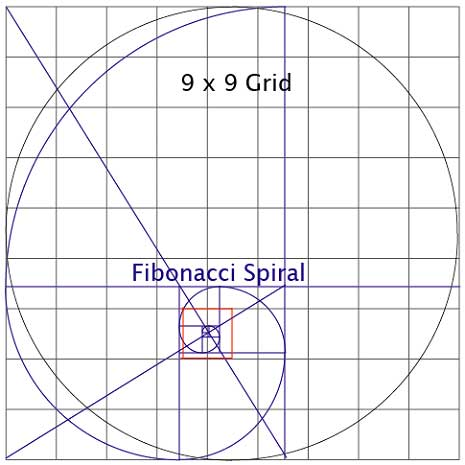Creating a 9×9 square grid equal to 9 base lengths of Khafre (in black) and dividing it at its Phi ratio point, (in blue), then drawing a Fibonacci spiral we associate the location of the Sphinx to the geometry. Notice the orange square, it is 1/64th of the 8×8 grid that is associated with the Sphinx, (maybe the “missing 1/64th of the Eye of Ra?) If we recall that Menkaure’s Angle #1 gave us the number for its volume of 7,997,790 and we have accounted for the three 7s, we need to explain the three 9s. I hypothesize that our three 9s are 9 + 9 + 9 = 27 and the Sphinx length is 1/27th of the diameter of the main circle. (I will cover in detail the exact circle diameter of 6355.554 ft equal to 3699 Royal Cubits, 20.61818 inches at another point). Here is the calculation. 6355.554 ft ÷ 27 = 235.390889 ft.

235.390889 ft x 12 = 2,824.69067 ÷ 20.61818 (Royal Cubit inches) = 137 Royal Cubits, proposed length of the Sphinx. Next let’s take a closer look at the (missing?) 1/64th square of the 8×8 grid in orange overlaid on the Giza image.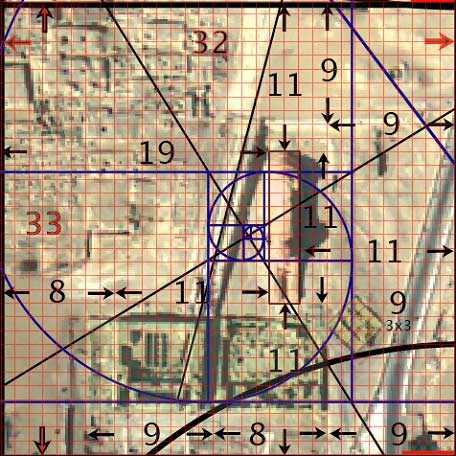Remember our main circle circumference was divided by 8 at 45°, 9 at 40° and 11 at 32.7272°. We now address the number 11. Take a moment and study this graphic there is quite a bit going on here. First I hypothesized the length of the Sphinx as 1/27th (235.390889 ft) of the overall circle diameter of 6355.554 ft. This positions the Sphinx’s length in the center 1/3 of the square, 706.1727 ft ÷ 3 = 235.390889 ft.

As 11 divides the circles circumference 32.7272° we are no longer working with whole numbers, if we use the two whole numbers on either side 33 and 32 then divide our 706.554 ft square into 33 vertical divisions and 32 horizontal divisions astonishing alignments are revealed. We assign each rectangle which has a ratio of 33/32 as 1 and count the rectangles in from the edges of our 706.554 ft square we see that the Sphinx is 11 in length, 2 in width and 3 height (706.554 ÷ 32 = 22.0798125 x 3 = 66.2394 ft). It is located 11 down from the top (West) edge, 11 in from the right (North) edge of the square, 11 up from the bottom (East) edge, and 19 (11+8) from the left (South) edge of the square. Take notice of the cut-out or “basin” that the Sphinx is enclosed by, 9 down, and 9 over, notice the Temple (shaded in light green) is 7 wide by 17 (9+8) in length. The exclamation point here is the 3×3 (shaded lightly in yellow) on a 45° angle to the grid is a ruin whose dimensions fit with a 3×3 rectangle with a ratio of 33/32, this acts as a legend or key on a blueprint indicating a particular scale or grid. I don’t know exactly what the chances are that all the geometrical and mathematical alignments being “coincidental” are but I do not consider myself a “coincidence theorist” do you?I tried many different grid divisions in an attempt to align all these structures and none and I mean none of them came close to explaining the layout of these structures. The division of this 706.1727 ft x 706.1727 ft by 33 vertically and 32 horizontally fit exactly into the hypothesis. In my opinion this is very compelling evidence for the Giza Template hypothesis. This ties the Sphinx in with the Giza Plateau and it’s pyramids in a logical and coherent manner. There are those who will argue this point, but to them I say, count the coincidences.

## Locating Giza Image on the 8×8 Number Grid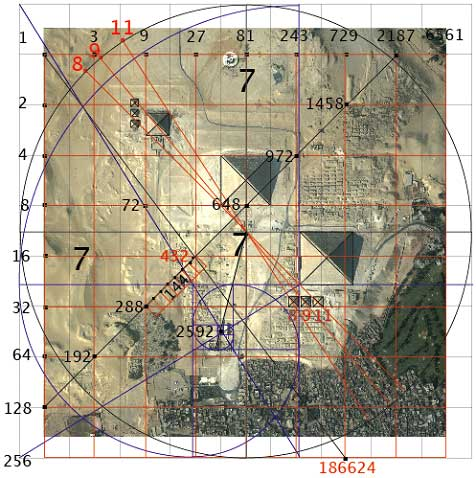Overlaying the 8×8 number grid over the Giza image reveals extremely interesting results. Take notice of the angled blue line beginning from the upper left corner of the 9×9 grid down to the Phi ratio division line at the bottom edge of the square. The line intersects an 8×8 grid intersection at 9 over at the top and 8 down the left side equaling 72 on the grid and at the exact center of our 1/64th square of 706.554 ft at 48 down and 54 across the top equaling 2592(0). If we consider the number 72 and 2592(0) as Root numbers of the Great year, 25920 years and 72 years for 1° of precession, we can use the number 144 to correct the Root to exact duration of years we currently are experiencing the rate of precession expressed in this equation: 25,920 – 144 = 25,776 ÷ 360 = 71.6 years per 1° of precession!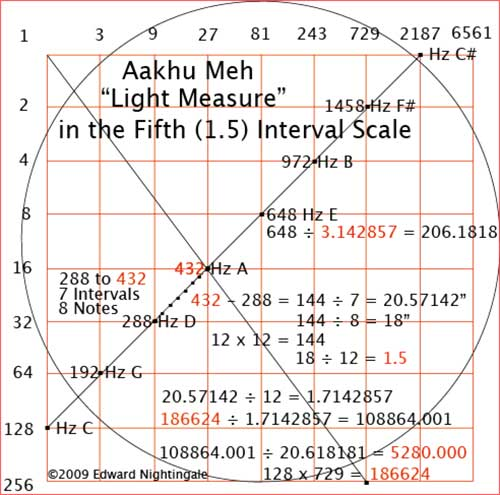Above is the measuring system found in the fifth interval scale. There are 3 intervals to arrive at 432; 7 also divide each interval. Starting with128 and ending with 432 there are 21 intervals (3 7s). There are three self-confirming equations to find the Root Cubit of 20.57142 using our numbers of 7, 8, 9, and 11, they are:
432 ÷ 21 = 20.57142
432 – 288 = 144 ÷ 7 = 20.57142
72 ÷ 11 = 6.545454 x 3.142857 (Pi 22/7)= 20.57142

The number 648 one interval up the main scale from 432 can be divided to find the Royal Cubit by moving a decimal point one place; 648 ÷ 3.142857 = 206.1818 to 20.61818.

Determining the Royal Cubit of 20.61818 from the Root Cubit of 20.57142 is accomplished with the following equation:

Adding the 440th part of 20.57142 to 20.57142
20.571428 ÷ 440 = .046753245 + 20.571428 = 20.61818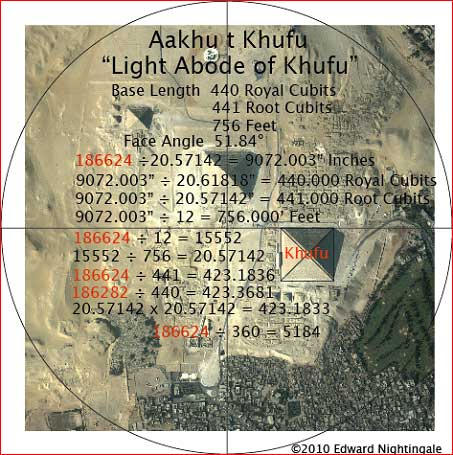### Books by Edward Nightingale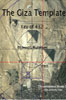#### The Giza Template

The equations in the graphic above I believe are what the original designers intended to encode within the Pyramid of Khufu. This in no way is stating that is the only function of Khufu, quite the contrary; I feel the encoded math, science and astronomical information are there to help us understand the real science and reason for constructing these enigmatic structures.

The intent of the original designers were multifaceted, I am pointing to one of those facets in the two articles I have submitted here for critique. There has been disagreement with some of the geometry to which I will state once again, the simple geometry will approximate and the encoded number system and mathematics allows the exact numbers to be determined.

I did not and could not have invented all of the information I have presented here; it was re-discovered by using simple logic, simple tools, and the simple truth, and that is exactly what I feel the builders intended.